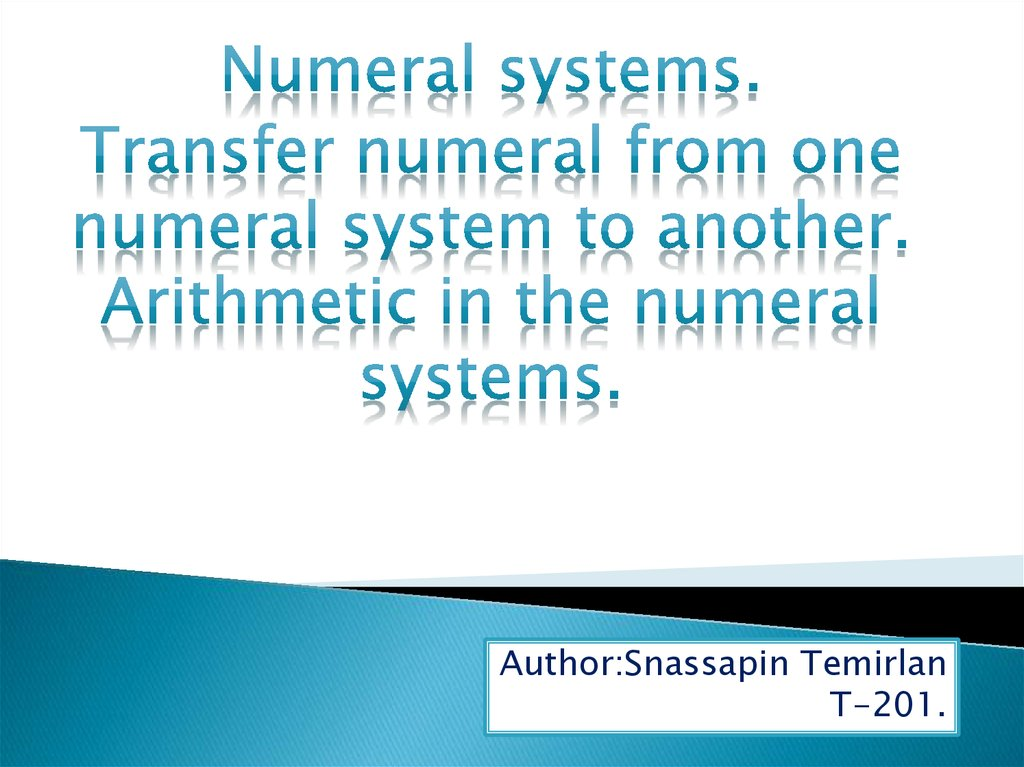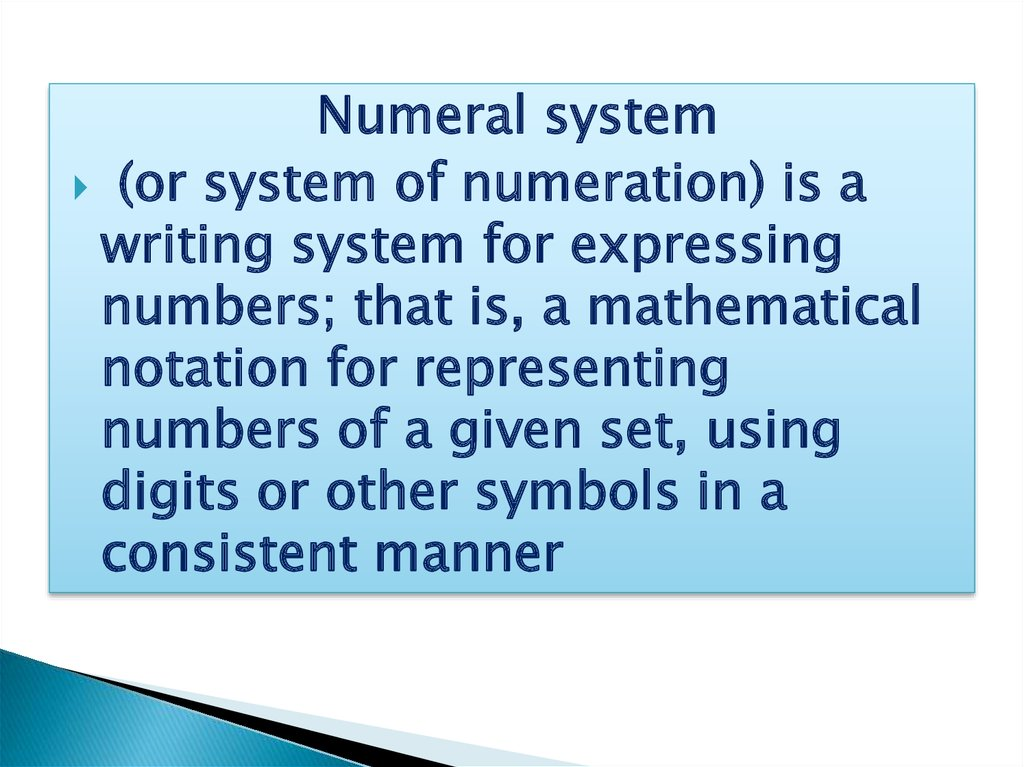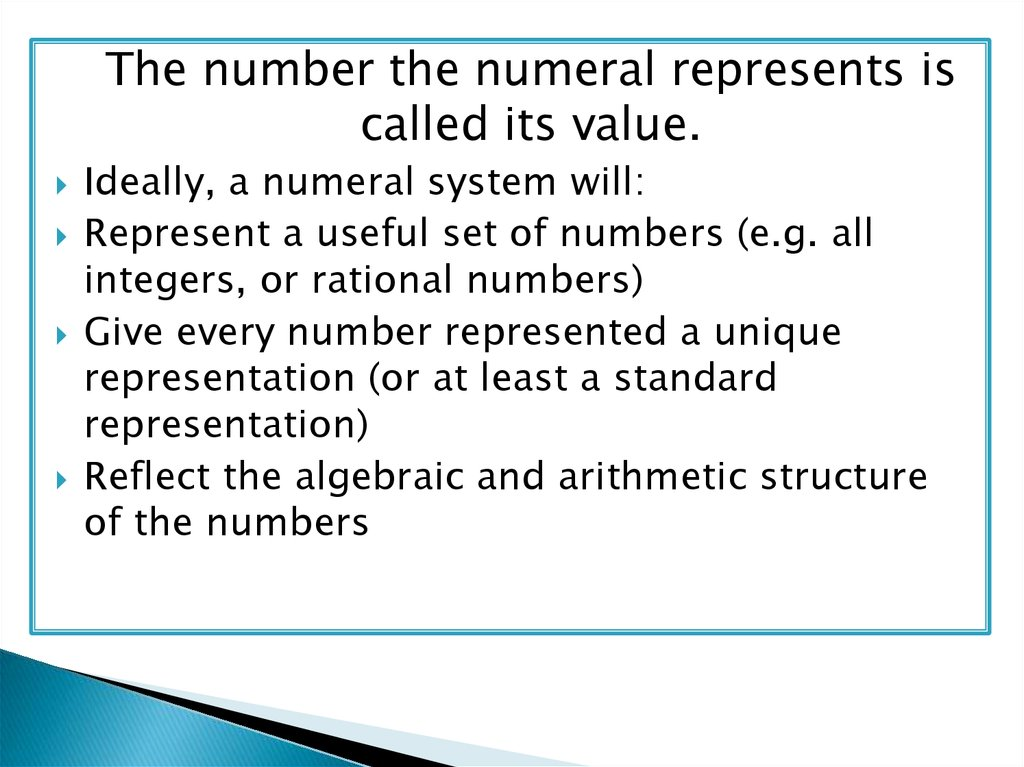# Numeral system

## 1.

Author:Snassapin Temirlan
T-201.

## 2.

Numeral system
(or system of numeration) is a
writing system for expressing
numbers; that is, a mathematical
notation for representing
numbers of a given set, using
digits or other symbols in a
consistent manner

## 3.

The number the numeral represents is
called its value.
Ideally, a numeral system will:
Represent a useful set of numbers (e.g. all
integers, or rational numbers)
Give every number represented a unique
representation (or at least a standard
representation)
Reflect the algebraic and arithmetic structure
of the numbers

## 4. Main numeral systems

The most commonly used system of
numerals is the Hindu–Arabic numeral
system. Two Indian mathematicians are
credited with developing it. Aryabhata of
Kusumapura developed the place-value
notation in the 5th century and a century later
Brahmagupta introduced the symbol for zero.

## 5. Decimal Numbers

Decimal numbers (base 10)
◦ Represented using 10 numerals: 0, 1, 2, 3, 4, 5, 6,
7, 8, 9
Each position represents a power of 10:
◦ 401= 4*102+ 0*101 + 1*100 = 400+ 1
◦ 130= 1*102 + 3*101+0*100 = 100 + 30
◦ 9786= 9*103 + 7*102 + 8*101 + 6*100=
= 9*1000 +7*100 + 8*10 + 6*1

## 6. Binary Numeral System

Binary numbers are represented by sequence
of bits (smallest unit of information – 0 or 1)
◦ Bits are easy to represent in electronics
1
1
1
1
0
0
1
0
0
0
1
1
1
1
1
1
0
0
1
0
0
0
1
0
1
1
1
1
0
1
1
0

## 7. Binary Numbers

Binary numbers (base 2)
◦ Represented by 2numerals: 0and 1
Each position represents a power of 2:
◦ 101b= 1*22 + 0*21 + 1*20 = 100b + 1b = 4+1= 5
◦ 110b = 1*22 + 1*21 + 0*20 = 100b + 10b = 4+2=6
◦ 110101b= 1*25 + 1*24 + 0*23 + 1*22 + 0*21+ 1*20=
= 32 + 16 + 4 + 1= = 53

## 8. How ComputersRepresent Text Data?

A text encoding is a system that uses binary
numbers (1and 0) torepresent characters
◦ Letters, numerals, etc.
In the ASCII encoding each character consists
of 8 bits (one byte) of data
◦ ASCII is used in nearly all personal computers
In the Unicode(UTF-16) encoding each
character consists of 16 bits (two bytes)
◦ Can represent many alphabets